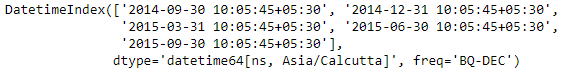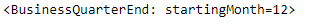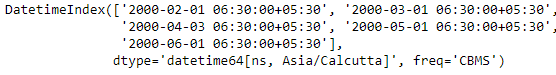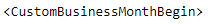Open in App
Not now

# Python | Pandas DatetimeIndex.freq

• Last Updated : 24 Dec, 2018

Python is a great language for doing data analysis, primarily because of the fantastic ecosystem of data-centric python packages. Pandas is one of those packages and makes importing and analyzing data much easier.

Pandas` DatetimeIndex.freq` attribute returns the frequency object if it is set in the DatetimeIndex object. If the frequency is not set then it returns None.

Syntax: DatetimeIndex.freq

Return: frequency object

Example #1: Use `DatetimeIndex.freq` attribute to find the frequency for the given DatetimeIndex object.

 `# importing pandas as pd``import` `pandas as pd`` ` `# Create the DatetimeIndex``# Here 'BQ' represents Business quarter frequency``didx ``=` `pd.DatetimeIndex(start ``=``'2014-08-01 10:05:45'``, freq ``=``'BQ'``, ``                               ``periods ``=` `5``, tz ``=``'Asia/Calcutta'``)`` ` `# Print the DatetimeIndex``print``(didx)`

Output :Now we want to find the value of frequency for the given DatetimeIndex object.

 `# find the value of frequency``didx.freq`

Output :As we can see in the output, the function has returned a frequency object for the given DatetimeIndex object.

Example #2: Use `DatetimeIndex.freq` attribute to find the frequency for the given DatetimeIndex object.

 `# importing pandas as pd``import` `pandas as pd`` ` `# Create the DatetimeIndex``# Here 'CBMS' represents custom business month start frequency``didx ``=` `pd.DatetimeIndex(start ``=``'2000-01-10 06:30'``, freq ``=``'CBMS'``,``                               ``periods ``=` `5``, tz ``=``'Asia/Calcutta'``)`` ` `# Print the DatetimeIndex``print``(didx)`

Output :Now we want to find the value of frequency for the given DatetimeIndex object.

 `# find the value of frequency``didx.freq`

Output :As we can see in the output, the function has returned a frequency object for the given DatetimeIndex object. The didx DatetimeIndex object is having custom business month start frequency.

My Personal Notes arrow_drop_up